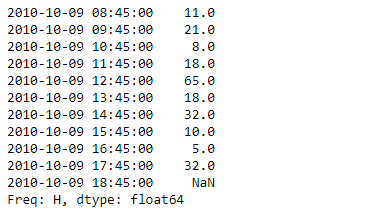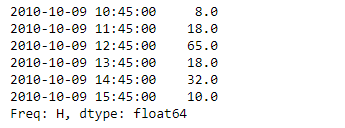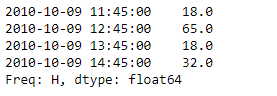# Python | Pandas Series.between_time()

• Last Updated : 17 Feb, 2019

Pandas series is a One-dimensional ndarray with axis labels. The labels need not be unique but must be a hashable type. The object supports both integer- and label-based indexing and provides a host of methods for performing operations involving the index.

Pandas` Series.between_time()` function select values between particular times of the day (e.g., 9:00-9:30 AM). By setting start_time to be later than end_time, you can get the times that are not between the two times.

Syntax: Series.between_time(start_time, end_time, include_start=True, include_end=True, axis=None)

Parameter :
start_time : datetime.time or string
end_time : datetime.time or string
include_start : boolean, default True
include_end : boolean, default True
axis : {0 or ‘index’, 1 or ‘columns’}, default 0

Returns : values_between_time : same type as caller

Example #1: Use `Series.between_time()` function to return the values lying in the given time duration.

 `# importing pandas as pd ` `import` `pandas as pd ` ` `  `# Creating the Series ` `sr ``=` `pd.Series([``11``, ``21``, ``8``, ``18``, ``65``, ``18``, ``32``, ``10``, ``5``, ``32``, ``None``]) ` ` `  `# Create the Index ` `index_ ``=` `pd.date_range(``'2010-10-09 08:45'``, periods ``=` `11``, freq ``=``'H'``) ` ` `  `# set the index ` `sr.index ``=` `index_ ` ` `  `# Print the series ` `print``(sr) `

Output :Now we will use `Series.between_time()` function to return the values lying in the given time duration.

 `# return values between the passed time duration ` `result ``=` `sr.between_time(start_time ``=` `'10:45'``, end_time ``=` `'15:45'``) ` ` `  `# Print the result ` `print``(result) `

Output :As we can see in the output, the `Series.between_time()` function has successfully returned the values lying in the given time duration.

Example #2 : Use `Series.between_time()` function to return the values lying in the given time duration. Skip the values corresponding to the start and end time.

 `# importing pandas as pd ` `import` `pandas as pd ` ` `  `# Creating the Series ` `sr ``=` `pd.Series([``11``, ``21``, ``8``, ``18``, ``65``, ``18``, ``32``, ``10``, ``5``, ``32``, ``None``]) ` ` `  `# Create the Index ` `index_ ``=` `pd.date_range(``'2010-10-09 08:45'``, periods ``=` `11``, freq ``=``'H'``) ` ` `  `# set the index ` `sr.index ``=` `index_ ` ` `  `# Print the series ` `print``(sr) `

Output :Now we will use `Series.between_time()` function to return the values lying in the given time duration. Skip the values corresponding to the start and end time.

 `# return values between the passed time duration ` `# skip the start and end time ` `result ``=` `sr.between_time(start_time ``=` `'10:45'``, end_time ``=` `'15:45'``, ` `                       ``include_start ``=` `False``, include_end ``=` `False``) ` ` `  `# Print the result ` `print``(result) `

Output :As we can see in the output, the `Series.between_time()` function has successfully returned the values lying in the given time duration. Notice the values corresponding to the start and end time has not been included.

My Personal Notes arrow_drop_up
Recommended Articles
Page :